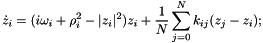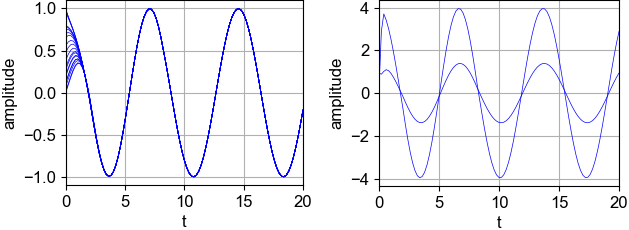pyclustering.nnet.fsync.fsync_network Class Reference

Model of oscillatory network that uses Landau-Stuart oscillator and Kuramoto model as a synchronization mechanism. More...Inheritance diagram for pyclustering.nnet.fsync.fsync_network:Collaboration diagram for pyclustering.nnet.fsync.fsync_network:

## Public Member Functions

def __init__ (self, num_osc, factor_frequency=1.0, factor_radius=1.0, factor_coupling=1.0, type_conn=conn_type.ALL_TO_ALL, representation=conn_represent.MATRIX)
Constructor of oscillatory network based on synchronization Kuramoto model and Landau-Stuart oscillator. More...

def simulate (self, steps, time, collect_dynamic=False)
Performs static simulation of oscillatory network. More...Public Member Functions inherited from pyclustering.nnet.network
def height (self)
Height of the network grid (that is defined by amout of oscillators in each column), this value is zero in case of non-grid structure. More...

def width (self)
Width of the network grid, this value is zero in case of non-grid structure. More...

def structure (self)
Type of network structure that is used for connecting oscillators.

def __init__ (self, num_osc, type_conn=conn_type.ALL_TO_ALL, conn_repr=conn_represent.MATRIX, height=None, width=None)
Constructor of the network. More...

def __len__ (self)
Returns size of the network that is defined by amount of oscillators.

def has_connection (self, i, j)
Returns True if there is connection between i and j oscillators and False - if connection doesn't exist. More...

def set_connection (self, i, j)
Couples two specified oscillators in the network with dynamic connections. More...

def get_neighbors (self, index)
Finds neighbors of the oscillator with specified index. More...

## Detailed Description

Model of oscillatory network that uses Landau-Stuart oscillator and Kuramoto model as a synchronization mechanism.

Dynamic of each oscillator in the network is described by following differential Landau-Stuart equation with feedback:Where left part of the equation is Landau-Stuart equation and the right is a Kuramoto model for synchronization. For solving this equation Runge-Kutta 4 method is used by default.

Example:

# Prepare oscillatory network parameters.
amount_oscillators = 3;
frequency = 1.0;
coupling_strength = 1.0;
# Create oscillatory network
oscillatory_network = fsync_network(amount_oscillators, frequency, radiuses, coupling_strength);
# Simulate network during 200 steps on 10 time-units of time-axis.
output_dynamic = oscillatory_network.simulate(200, 10, True); # True is to collect whole output dynamic.
# Visualize output result
fsync_visualizer.show_output_dynamic(output_dynamic);

Example of output dynamic of the network:Definition at line 166 of file fsync.py.

## ◆ __init__()

 def pyclustering.nnet.fsync.fsync_network.__init__ ( self, num_osc, factor_frequency = 1.0, factor_radius = 1.0, factor_coupling = 1.0, type_conn = conn_type.ALL_TO_ALL, representation = conn_represent.MATRIX )

Constructor of oscillatory network based on synchronization Kuramoto model and Landau-Stuart oscillator.

Parameters
 [in] num_osc (uint): Amount oscillators in the network. [in] factor_frequency (double|list): Frequency of oscillators, it can be specified as common value for all oscillators by single double value and for each separately by list. [in] factor_radius (double|list): Radius of oscillators that affects amplitude, it can be specified as common value for all oscillators by single double value and for each separately by list. [in] factor_coupling (double): Coupling strength between oscillators. [in] type_conn (conn_type): Type of connection between oscillators in the network (all-to-all, grid, bidirectional list, etc.). [in] representation (conn_represent): Internal representation of connection in the network: matrix or list.

Definition at line 206 of file fsync.py.

## ◆ simulate()

 def pyclustering.nnet.fsync.fsync_network.simulate ( self, steps, time, collect_dynamic = False )

Performs static simulation of oscillatory network.

Parameters
 [in] steps (uint): Number simulation steps. [in] time (double): Time of simulation. [in] collect_dynamic (bool): If True - returns whole dynamic of oscillatory network, otherwise returns only last values of dynamics.
Returns
(list) Dynamic of oscillatory network. If argument 'collect_dynamic' is True, than return dynamic for the whole simulation time, otherwise returns only last values (last step of simulation) of output dynamic.